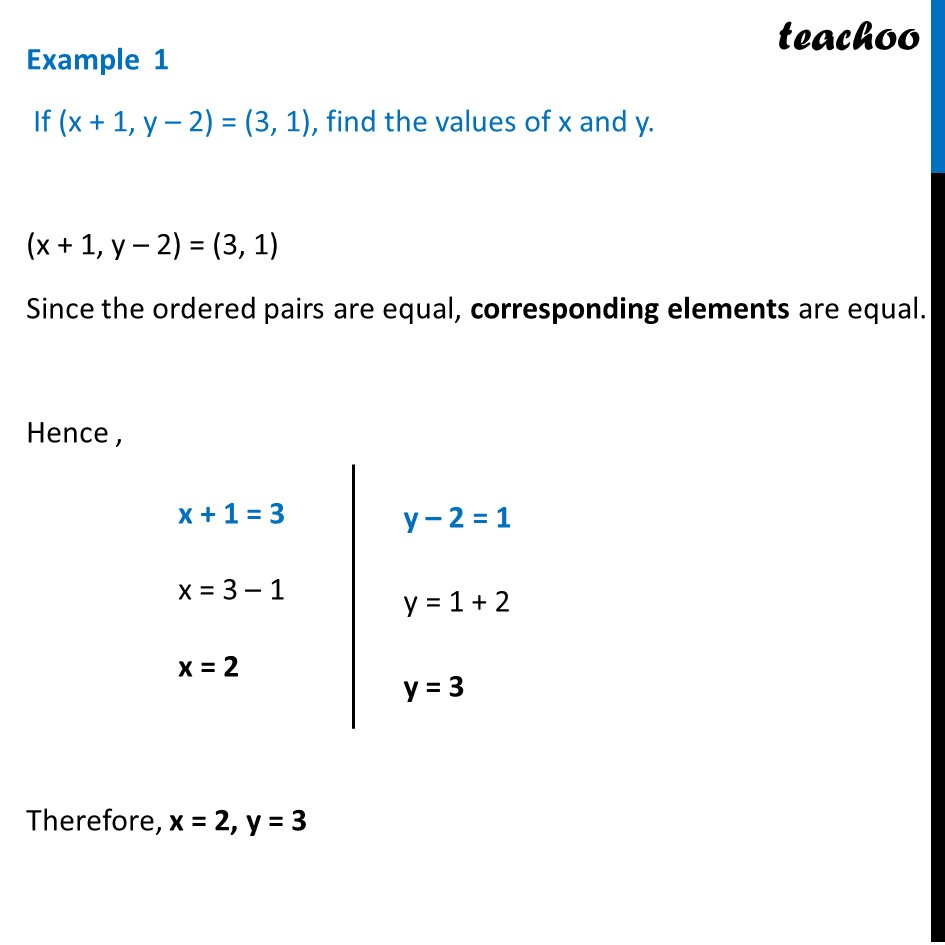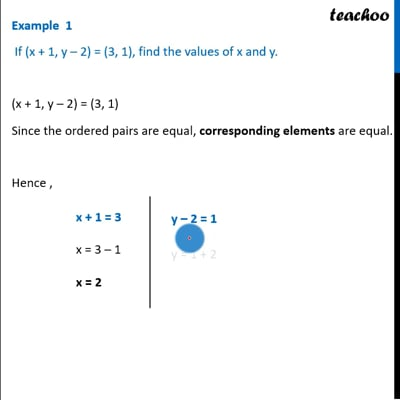Examples

Chapter 2 Class 11 Relations and Functions (Term 1)
Serial order wiseThis video is only available for Teachoo black users

### Transcript

Example 1 If (x + 1, y – 2) = (3, 1), find the values of x and y. (x + 1, y – 2) = (3, 1) Since the ordered pairs are equal, corresponding elements are equal. Hence , x + 1 = 3 x = 3 – 1 x = 2 y – 2 = 1 y = 1 + 2 y = 3 Therefore, x = 2, y = 3